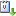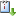Usted está aquí: Algebraic and combinatorial decompositions of Fuchsian groups 1

# Algebraic and combinatorial decompositions of Fuchsian groups 1

Ponente: Daniel Labardini
Institución: IM-UNAM
Tipo de Evento: Investigación, Formación de Recursos Humanos
Cuándo 17/02/2020 de 16:30 a 18:00 Salón 1 de seminariosvCaliCal

The discrete subgroups of PSL_2(R) are often called 'Fuchsian groups'. For Fuchsian groups \Gamma whose action on the hyperbolic plane H is free, the orbit space H/\Gamma has a canonical structure of Riemann surface with a hyperbolic metric, whereas if the action of \Gamma is not free, then H/\Gamma has a structure of 'orbifold'. In the former case, there is a direct and very clear relation between \Gamma and the fundamental group \pi_1(H/\Gamma,x): a theorem of the theory of covering spaces states that they are isomorphic. When the action of \Gamma is not free, the relation between \Gamma and \pi_1(H/\Gamma,x) is subtler. A 1968 theorem of Armstrong states that there is a short exact sequence 1->E->\Gamma->\pi_1(H/\Gamma,x)->1, where E is the subgroup of \Gamma generated by the elliptic elements. For \Gamma finitely generated, non-elementary and with at least one parabolic element, I will present full algebraic and combinatorial decompositions of \Gamma in terms of \pi_1(H/\Gamma,x) and a specific finitely generated subgroup of E, thus improving Armstrong's theorem.

This talk is based on an ongoing joint project with Sibylle Schroll and Yadira Valdivieso-Díaz that aims at describing the bounded derived categories of skew-gentle algebras in terms of curves on surfaces with orbifold points of order 2.